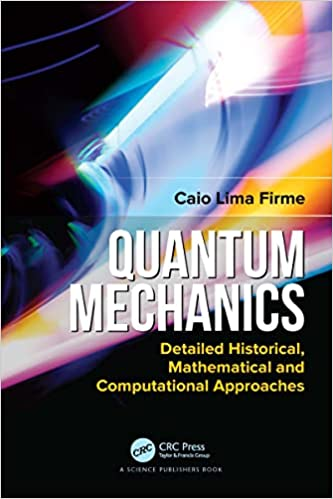# Quantum Mechanics Detailed Historical, Mathematical and Computational ApproachesEnglish | 2022 | ISBN: 0367506335 | 462 pages | True PDF EPUB | 25.39 MB
Main features: i A different approach for teaching Quantum Mechanics encompassing old quantum mechanics, matrix mechanics and wave mechanics in a historical perspective which helps to consolidate most important concepts of Quantum Mechanics; ii Original information from the most important papers of Quantum Mechanics; iii Derivation of all important equations of Quantum Mechanics, for example, Heisenberg’s uncertainty principle, de Broglie’s wave-particle duality, Schrödinger’s wave equation, etc., showing their interrelations through Dirac’s equations and other applications of matrix and wave mechanics; iv Comprehensive mathematical support for the understanding of Quantum Mechanics; derivation of all equations make reading easier; v The illustrations of the book cover examples, exercises and do-it-yourself activities; vi Fundamentals of Fortran and numerical calculation along with the source codes for numerical solutions of several mathematical and quantum problems. All source codes are in the author’s site: (https://www.fortrancodes.com/); vii Chapters devoted to linear algebra and differential equations applied to quantum mechanics and their numerical solutions; viii Complete solution for the one-electron and two-electron problems using Schrödinger’s time independent equation along with their source codes. Derivation of all important equations of Quantum Mechanics, for example, Heisenberg’s uncertainty principle, de Broglie’s wave-particle duality, Schrödinger’s wave equation, etc, showing their interrelations through Dirac’s equations and other applications of matrix and wave mechanics;